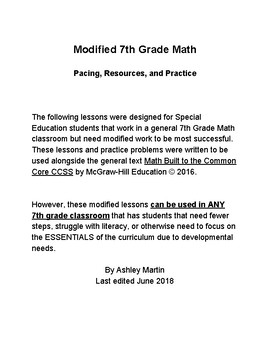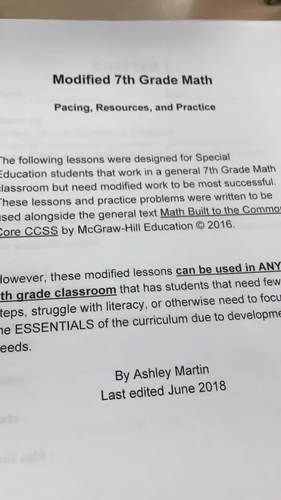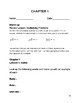# 7th Grade Math Ch 1 RP Modified Lessons 1-9Subject
Resource Type
File Type

PDF

(389 KB|20 pages)
Standards
• Product Description
• Standards
3 weeks of 7th grade Unit 1 RP ESSENTIALS for students that struggle. Any teacher that wants to scaffold the 7th grade curriculum or modify it for a struggling student can use this scaffolded, 20 page practice of 7th grade Ratios and Proportional Reasoning Skills. This practice was first written with the intention of inviting very low skill, special ed students to be part of the 7th grade content rather than cast aside on a computer like many kids on different tracks get relegated, but it has proven to help roughly 30% of my students access an otherwise very frustrating 7th grade Math year. This is only Chapter 1 in the year long series I am uploading.
Use proportional relationships to solve multistep ratio and percent problems. Examples: simple interest, tax, markups and markdowns, gratuities and commissions, fees, percent increase and decrease, percent error.
Explain what a point (𝘹, 𝘺) on the graph of a proportional relationship means in terms of the situation, with special attention to the points (0, 0) and (1, 𝘳) where 𝘳 is the unit rate.
Represent proportional relationships by equations. For example, if total cost 𝘵 is proportional to the number 𝘯 of items purchased at a constant price 𝘱, the relationship between the total cost and the number of items can be expressed as 𝘵 = 𝘱𝘯.
Identify the constant of proportionality (unit rate) in tables, graphs, equations, diagrams, and verbal descriptions of proportional relationships.
Decide whether two quantities are in a proportional relationship, e.g., by testing for equivalent ratios in a table or graphing on a coordinate plane and observing whether the graph is a straight line through the origin.
Total Pages
20 pages
Not Included
Teaching Duration
3 Weeks
Report this Resource to TpT
Reported resources will be reviewed by our team. Report this resource to let us know if this resource violates TpT’s content guidelines.